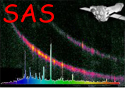XMM-Newton Science Analysis System

lcplot (lcplot-1.22.1) [xmmsas_20211130_0941-20.0.0]

# Algorithm

```   Subroutine lcplot

Recover parameters (File names, Device type, Binning factor, Ouput file)

Read source time series FITS file :
Get dataset and table.
Check important keyword consistency (notably TSTART and TIMEDEL).
Call a warning or error if necessary.

Recover all light curves included in table (in PPS use, 3 for EPIC, 1 for OM) :
Net source rates and errors & background rates and errors are recorded in arrays
of dimension Nlightcurve * Nbins.

Open plot : create view surface in specified device format (check its validity).

For each light curve :

Delete gaps in data (when the IEEE NaN constant is found).

Increase binsize if necessary to limit the number of points to 500.

Rebin rate and error columns by binsize

Create a labelled plot page separated into two panels in which time is graduated
on X-axis (see Comments), and count rates on Y-axis (in counts/sec).

Draw net source and background time series on different panels.

Reconstruct net source counts and total counts rebinned :

Perform variability tests on rebinned net source counts :
Calculate mean count rate and variance.
Test the null hypothesis (the source is not variable) by :
- Processing a Chi Square fit between the count distribution and a
constant distribution whose value is equal to the observed mean (statistic
and probability are computed).
- Processing a Kolmogorov-Smirnov test between the cumulative probability
functions of the observed count distribution and a theorical Gaussian
distribution whose mean and variance are both equal to the observed mean
count.
End for

Write variability test results on a single plot page.

Release memory.

Close plot.

End subroutine  lcplot
```

XMM-Newton SOC -- 2021-11-30